MAHARASHTRA

XII (12) HSC

XI (11) FYJC
X (10) SSC

### EX. NO. 1.4, RELATION AND FUNCTIONS, 10TH NEW SYLLABUS, Tamil Nadu, TN,

1. Determine whether the graph given below represent functions. Give reasons for your answers concerning each graph.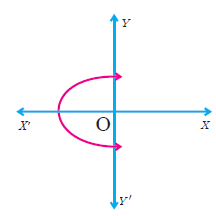Solution :
Since the graph intersects the vertical line (y-axis) at two points, it is not a function.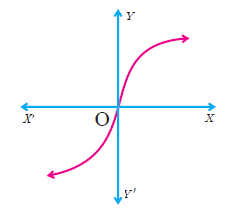Solution :
The given graph intersects the vertical line (y-axis) at one point. It is a function.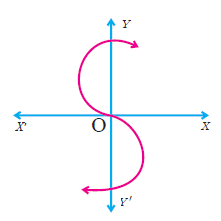Solution :
The graph intersects the y-axis at three points, hence it is not a function.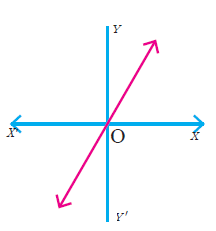Solution :
The graph intersects the vertical line at most one point. Hence it is a function.

2. Let f : A -> B be a function defined by
f (x) =  (x/2) - 1,
where A = {2, 4, 6, 10, 12}, B = {0, 1, 2, 4, 5, 9} .
Represent f by
(i) set of ordered pairs;
(ii) a table;
(iii) an arrow diagram;
(iv) a graph
Solution :
(i) set of ordered pairs
f (x) =  (x/2) - 1

 x = 2 f(2) = (2/2) - 1  = 1 - 1 f(2) = 0 x = 4 f(4) = (4/2) - 1  = 2 - 1 f(4) = 1 x = 6 f(6) = (6/2) - 1  = 3 - 1 f(6) = 2 x = 10 f(10) = (10/2) - 1  = 5 - 1 f(10) = 4 x = 12 f(12) = (12/2) - 1  = 6 - 1 f(12) = 5

Set of ordered pairs  = {(2, 0) (4, 1) (6, 2) (10, 4) (12, 5)}
(ii) a table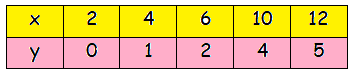(iii) an arrow diagram;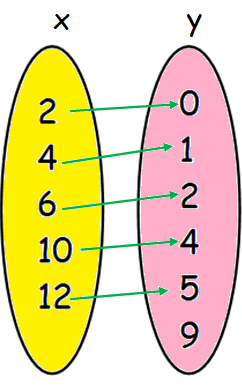(iv) a graph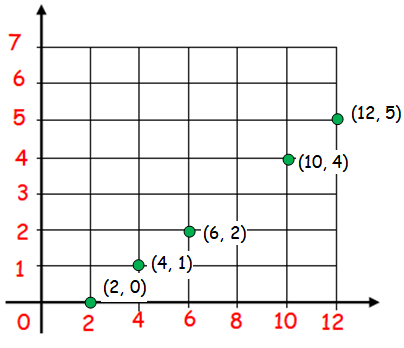3. Represent the function f = {(1, 2),(2, 2),(3, 2),(4, 3),(5, 4)} through (i) an arrow diagram (ii) a table form (iii) a graph
Solution :
(i) an arrow diagram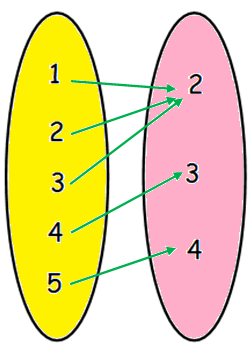(ii) a table form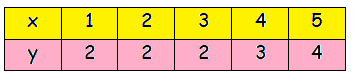(iii) a graph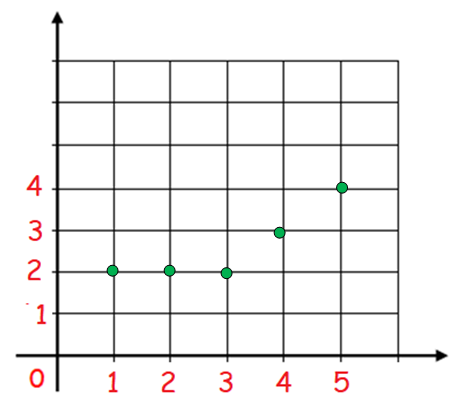4. Show that the function f : N -> N defined by f (x) = 2x – 1 is one-one but not onto
Solution :
If for all a1, a2 ∈ A, f(a1) = f(a2) implies a1 = a2 then f is called one – one function.
Let x, y ∈ N, f(x)  = f(y)
f(x)  = 2x - 1  -----(1)
f(y)  = 2y - 1  -----(2)
(1)  = (2)
2x - 1  = 2y - 1
2x  = 2y
x  = y
Hence the function is one to one.
It is not onto :
If co-domain of the function = range of function, then the function is said to be onto.
Even numbers in the co-domain are not associated with the elements of domain. Hence it is not onto.

5. Show that the function f : N -> N defined by f (m) = m2 + m + 3 is one-one function.
Solution :
Let x, y ∈ N, f(x)  = f(y)
f (m) = m2 + m + 3
f(x)  = x2 + x + 3  -----(1)
f(y)  = y2 + y + 3  -----(2)
(1)  = (2)
x2 + x + 3  = y2 + y + 3
x2 + x  = y2 + y
x2 - y2 + x - y = 0
(x + y) (x - y) + (x - y)  = 0
(x - y) (x + y + 1)  = 0
x - y  = 0
x  = y
Hence it is one to one function.

6. Let A = {1, 2, 3, 4} and B = N . Let f : A -> B be defined by f (x) = x3 then, (i) find the range of f (ii) identify the type of function
Solution :
Given that :
f (x) = x3

 f (x) = x3 x = 1 f (1) = 13  = 1 f (x) = x3 x = 2 f (2) = 23  = 8 f (x) = x3 x = 3 f (3) = 33  = 27 f (x) = x3 x = 4 f (4) = 43  = 64

Range of f  = {1, 8, 27, 64}
Every element in A has associated with different elements of B. Hence it is one to one.

7. In each of the following cases state whether the function is bijective or not. Justify your answer.
(i) f : R -> R defined by f (x) = 2x +1
Solution :
Testing whether it is one to one :
If for all a1, a2 ∈ A, f(a1) = f(a2) implies a1 = a2 then f is called one – one function.
Let x, y ∈ R, f(x)  = f(y)
f(x)  = 2x + 1  ------(1)
f(y)  = 2y + 1  ------(2)
(1) = (2)
2x + 1  = 2y + 1
2x  = 2y
x  = y
So, it is one to one.
Testing whether it is onto :
Range of f = co-domain
If f : A -> B is an onto function then, the range of f = B . That is, f(A) = B.
Let x ∈ A, y ∈ B and x, y ∈ R. Then, x is pre-image and y is image.
Then, we have
y  = 2x + 1
Solve for x.
x  = (y - 1) /2
Here, y is a real number. When we subtract 1 from a real number and the result is divided by 2, again it is a real number.
For every real number of y, there is a real number x.
So, range of f(x) is equal to co-domain.
It is onto function.
Hence it is bijective function.
(ii) f : R -> R defined by f (x) = 3 – 4x2
Solution :
Testing whether it is one to one :
If for all a1, a2 ∈ A, f(a1) = f(a2) implies a1 = a2 then f is called one – one function.
Let x, y ∈ R, f(x)  = f(y)
f(x)  = 3 – 4x2  ------(1)
f(y)  = 3 – 4y2  ------(2)
(1) = (2)
3 – 4x2  =  3 – 4y2
-4x2  = –4y2
x2 - y2 =  0
(x - y)(x + y) =  0
x - y = 0  (or) x + y =  0
x = y (or) x = -y
It is not one to one.Hence it is not bijective function.

8. Let A = {−1, 1}and B = {0, 2} . If the function f : A -> B defined by f(x) = ax + b is an onto function? Find a and b.
Solution :
f(x) = ax + b
f(-1) = a(-1) + b  = 0
-a + b  = 0 ------(1)
f(1) = a(1) + b  = 2
a + b  = 2 ------(2)
(1) + (2)
2b  = 2
b  = 1
By applying the value of b in (1), we get
-a + 1  = 0
-a  = -1
a  = 1
Hence the values of a and b are 1 and 1 respectively.
9. If the function f is defined by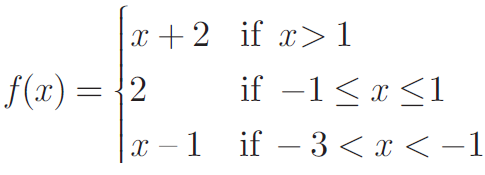find the values of
(i) f (3) (ii) f (0) (iii) f (−1.5) (iv) f (2)+ f (−2)
Solution :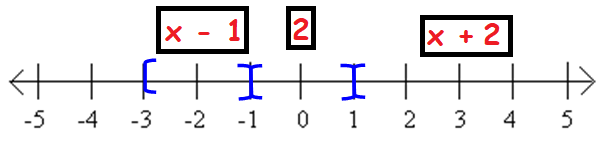(i)  f(3)
Instead of x, we have 3. So we have to choose the function f(x)  = x + 2
f(3)  = 3 + 2
f(3)  = 5
(ii)  f(0)
0 lies between -1 and 1. So, the answer is 2.
(iii) f (−1.5)
f(x)  = x - 1
f(-1.5)  = -1.5 - 1
f(-1.5)  = -2.5
(iv) f (2)+ f (−2)
f (2) + f (−2)  = 4 + (-3) = 1

10. A function f : [−5,9] -> R is defined as follows: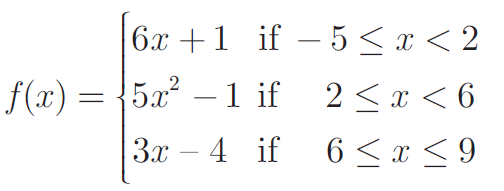Find (i) f (−3) + f (2)
(ii) f (7) - f (1)
(iii) 2f (4) + f (8)
(iv)  [2f(-2) - f(6)] / [f(4) + f(-2)]
Solution :
(i) f (−3) + f(2)
f(x)  = 6x + 1 for f(-3) and f(x)  = 5x2 - 1 for f(2)
f (−3) + f(2)  = -17 + 19
f (−3) + f(2)  = 2
(ii) f (7) - f (1)
f(x)  = 3x - 4 for f(7) and f(x)  = 6x + 1 for f(1)
f (7) - f (1)  = 17 - 7 = 10
(iii) 2f (4) + f (8)
f(x)  = 5x2 - 1 for f(4)  and f(x) = 3x - 4 for f(8)
2f (4) + f (8)  = 2(79) + 20
= 158 + 20
2f (4) + f (8)  = 178
(iv)  [2f(-2) - f(6)] / [f(4) + f(-2)]
f(x)  = 6x + 1 for f(-2) and f(x) = 3x - 4 for f(6)
[2f(-2) - f(6)] / [f(4) + f(-2)]  = [2(-11) - 14] / [79 + (-11)]
= (-22 - 14) / (79 - 11)
= -36/68
= -9/17

## PDF FILE TO YOUR EMAIL IMMEDIATELY PURCHASE NOTES & PAPER SOLUTION. @ Rs. 50/- each (GST extra)

SUBJECTS

HINDI ENTIRE PAPER SOLUTION

MARATHI PAPER SOLUTION
SSC MATHS I PAPER SOLUTION
SSC MATHS II PAPER SOLUTION
SSC SCIENCE I PAPER SOLUTION
SSC SCIENCE II PAPER SOLUTION
SSC ENGLISH PAPER SOLUTION
SSC & HSC ENGLISH WRITING SKILL
HSC ACCOUNTS NOTES
HSC OCM NOTES
HSC ECONOMICS NOTES
HSC SECRETARIAL PRACTICE NOTES

2019 Board Paper Solution

HSC ENGLISH SET A 2019 21st February, 2019

HSC ENGLISH SET B 2019 21st February, 2019

HSC ENGLISH SET C 2019 21st February, 2019

HSC ENGLISH SET D 2019 21st February, 2019

SECRETARIAL PRACTICE (S.P) 2019 25th February, 2019

HSC XII PHYSICS 2019 25th February, 2019

CHEMISTRY XII HSC SOLUTION 27th, February, 2019

OCM PAPER SOLUTION 2019 27th, February, 2019

HSC MATHS PAPER SOLUTION COMMERCE, 2nd March, 2019

HSC MATHS PAPER SOLUTION SCIENCE 2nd, March, 2019

SSC ENGLISH STD 10 5TH MARCH, 2019.

HSC XII ACCOUNTS 2019 6th March, 2019

HSC XII BIOLOGY 2019 6TH March, 2019

HSC XII ECONOMICS 9Th March 2019

SSC Maths I March 2019 Solution 10th Standard11th, March, 2019

SSC MATHS II MARCH 2019 SOLUTION 10TH STD.13th March, 2019

SSC SCIENCE I MARCH 2019 SOLUTION 10TH STD. 15th March, 2019.

SSC SCIENCE II MARCH 2019 SOLUTION 10TH STD. 18th March, 2019.

SSC SOCIAL SCIENCE I MARCH 2019 SOLUTION20th March, 2019

SSC SOCIAL SCIENCE II MARCH 2019 SOLUTION, 22nd March, 2019

XII CBSE - BOARD - MARCH - 2019 ENGLISH - QP + SOLUTIONS, 2nd March, 2019

HSC Maharashtra Board Papers 2020

(Std 12th English Medium)

HSC ECONOMICS MARCH 2020

HSC OCM MARCH 2020

HSC ACCOUNTS MARCH 2020

HSC S.P. MARCH 2020

HSC ENGLISH MARCH 2020

HSC HINDI MARCH 2020

HSC MARATHI MARCH 2020

HSC MATHS MARCH 2020

SSC Maharashtra Board Papers 2020

(Std 10th English Medium)

English MARCH 2020

HindI MARCH 2020

Hindi (Composite) MARCH 2020

Marathi MARCH 2020

Mathematics (Paper 1) MARCH 2020

Mathematics (Paper 2) MARCH 2020

Sanskrit MARCH 2020

Important-formula

THANKS